Courses

# Analysis of Arches & Cables Notes | EduRev

## GATE : Analysis of Arches & Cables Notes | EduRev

The document Analysis of Arches & Cables Notes | EduRev is a part of the GATE Course Structural Analysis.
All you need of GATE at this link: GATE

Arches

Three Hinged Arches

1. Three Hinged Parabolic Arch of Span L and rise 'h' carrying a UDL over the whole span
Ds = 0
BMc = 0
H = wl2/8h
Mx = VAx - wx2/2 - Hy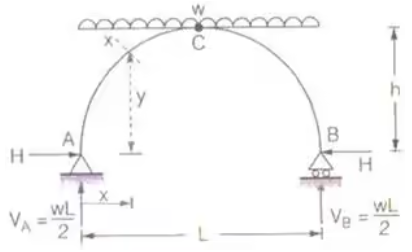where, H = Horizontal thrust
VA = Vertical reaction at A = wl/2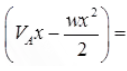Simply supported beam moment i.e., moment caused by vertical reactions.
Hy = H-moment
DS = Degree of static indeterminacy
BMC = Bending Moment at C.
2. Three Hinged Semicircular Arch of Radius R carrying a UDL over the whole span.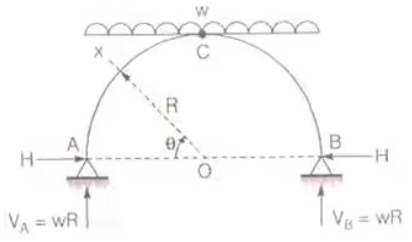H = wR/2
Mx = -wR2/2 [sin θ - sin2 θ]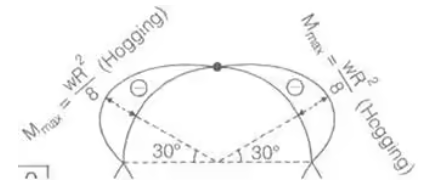Mmax = -wR2/8
BMc = 0
Point of contraflexure = 0
3. Three Hinged Parabolic Arch Having Abutments at Different Levels
(i) When it is subjected to UDL over whole span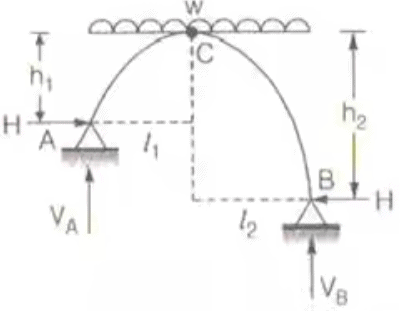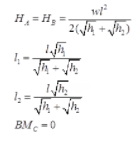(ii) When it is subjected to concentrated load W at crown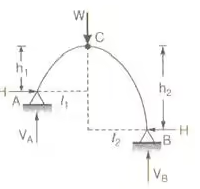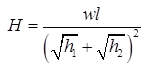4. Three Hinged Semicircular Arch Carrying Concentrated Load W at Crown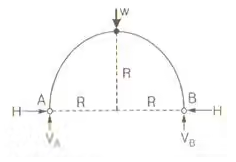H = VA = VB = W/2

Temperature Effect on Three Hinged Arches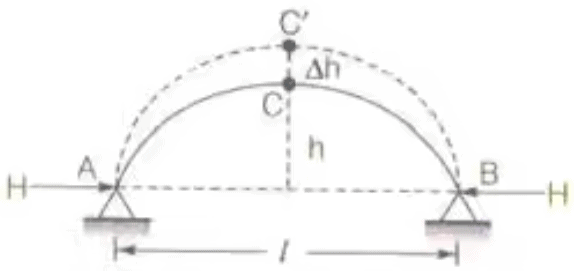1.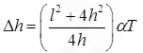Where, Δh = free rise in crown height
l = length of arch
h = rise of arch
α = coefficient of thermal expansion
T = rise in temperature in 0C
2. H α 1/h
Where, H = horizontal thrust
and h = rise of arch
3. % Decrease in horizontal thrust = δh/h x 100

Two Hinged Arches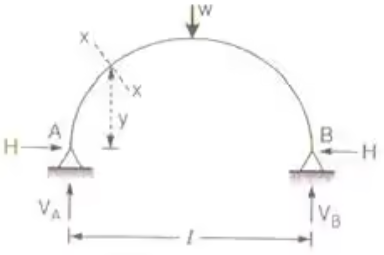Two hinged arch of any shape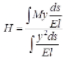DS = 1
Where, M = Simply support Beam moment caused by vertical force.

1. Two hinged semicircular arch of radius R carrying a concentrated load 'w' at the town.
H = w/π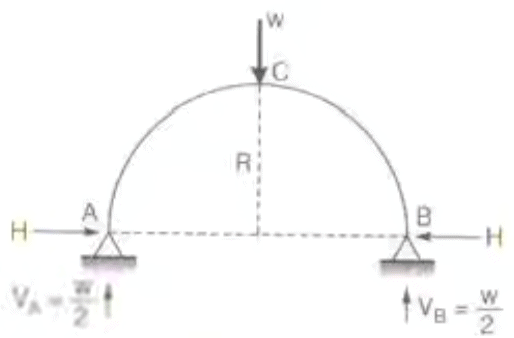Two Hinged Circular arch
2. Two hinged semicircular arch of radius R carrying a load w at a section, the radius vector corresponding to which makes an angle α with the horizontal.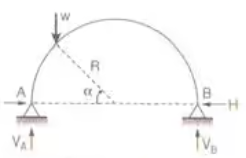Two Hinged Circular archH = w/π sin2 α
3. A two hinged semicircular arch of radius R carrying a UDL w per unit length over the whole span.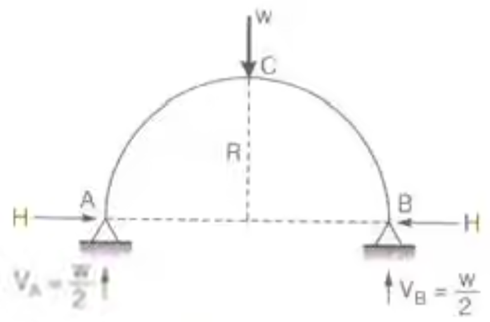Two Hinged Semicircular arch
4. A two hinged semicircular arch of radius R carrying a distributed load uniformly varying from zero at the left end to w per unit run at the right end.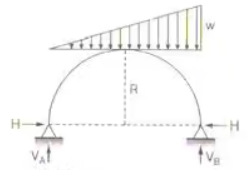Two Hinged Semicircular archH = 2/3.wR/π
5. A two hinged parabolic arch carries a UDL of w per unit run on entire span. If the span off the arch is L and its rise is h.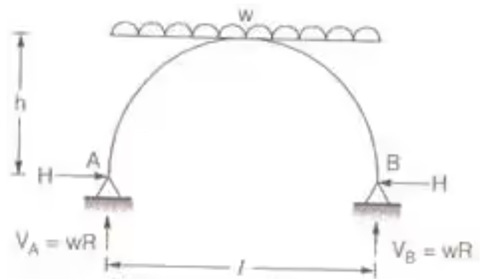Two Hinged parabolic arch
H = wl2/8h
6. When half of the parabolic arch is loaded by UDL, then the horizontal reaction at support is given by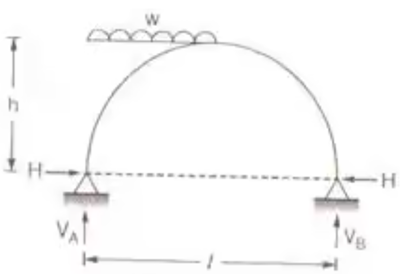Two Hinged parabolic arch
7. When two hinged parabolic arch carries varying UDL, from zero to w the horizontal thrust is given by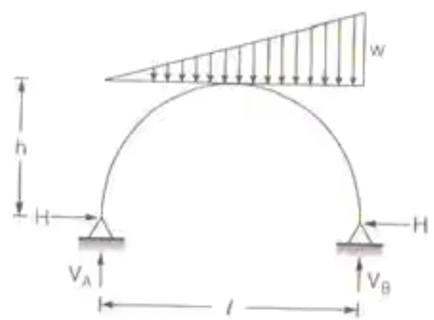Two Hinged parabolic arch
H = wl2/16h
8. A two hinged parabolic arch of span l and rise h carries a concentrated load w at the crown.
H = 25 wl/ 128 h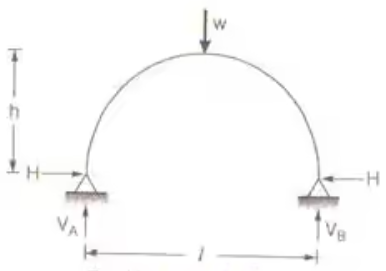Two Hinged parabolic arch
Temperature Effect on Two Hinged Arches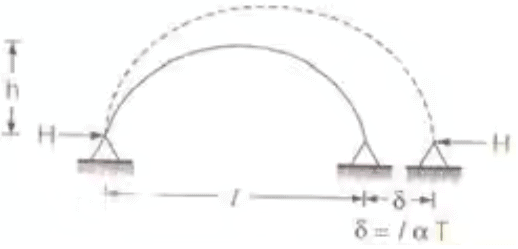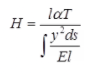1.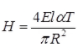where H = Horizontal thrust for two hinged semicircular arch due to rise in temperature by T 0C.
2.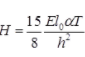where l0 = Moment of inertia of the arch at crown.
H = Horizontal thrust for two hinged parabolic arch due to rise in temperature T 0C.

Reaction Locus for a Two Hinged Arch

1. Two Hinged Semicircular Arch
Reaction locus is straight line parallel to the line joining abutments and height at πR/2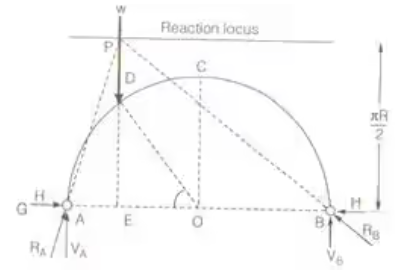2. Two Hinged Parabolic Arch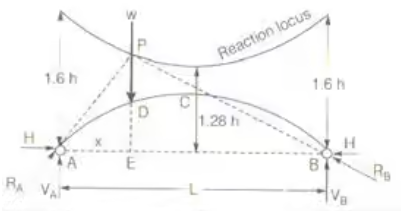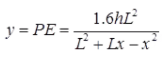Eddy's Theorem

Mxαy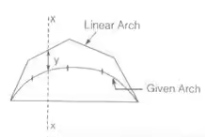where, MX = BM at any section
y = distance between given arch linear arch

Offer running on EduRev: Apply code STAYHOME200 to get INR 200 off on our premium plan EduRev Infinity!

## Structural Analysis

30 videos|122 docs|28 tests

,

,

,

,

,

,

,

,

,

,

,

,

,

,

,

,

,

,

,

,

,

;